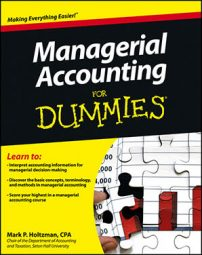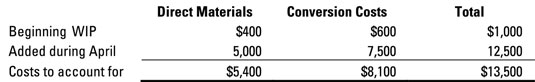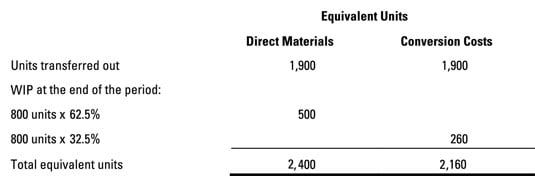##### Managerial Accounting For DummiesIn Part 3 of a cost of production report, you determine the total costs that were assigned to the department, including the cost of any beginning inventory; the cost of goods transferred from other departments; and any direct materials, direct labor, and overhead assigned directly to the department. You divide these total costs by the number of equivalent units the department produces to compute the cost per equivalent unit.

Consider the clown factory I discuss earlier in the chapter. The Balloon department started April with 200 units of beginning WIP, which cost \$400 for direct materials. Another \$5,000 worth of direct materials costs went into the department during April. Total direct materials costs equal \$5,400.

The Balloon department’s beginning WIP had \$600 worth of conversion costs. Another \$7,500 worth of conversion costs were allocated to the department during April, so total conversion costs came to \$8,100.## Divide by equivalent units to get cost per equivalent unit

To determine the cost per equivalent unit, divide total cost by the number of equivalent units, with respect to both direct materials and conversion costs:

Cost per equivalent unit = Total cost / Number of equivalent units

During April, the Balloon department finished 1,900 clowns and sent them to the next department. At the end of April, 800 clowns remained on the Balloon department’s assembly line. These clowns were 62.5 percent complete with respect to direct materials and 32.5 percent complete with respect to conversion costs.

To find the number of equivalent units, add the number of completed units transferred out to the number of equivalent units remaining in WIP at the end of the month. The Balloon department made 2,400 equivalent units with respect to direct materials and 2,160 equivalent units with respect to conversion costs.Finally, to figure out the equivalent cost per unit, divide total cost by total equivalent units. Direct materials cost \$2.25 per equivalent unit (\$5,400 ÷ 2,400 units). Conversion costs came to \$3.75 per equivalent unit (\$8,100 ÷ 2,160 units), so total cost per equivalent unit is \$6.